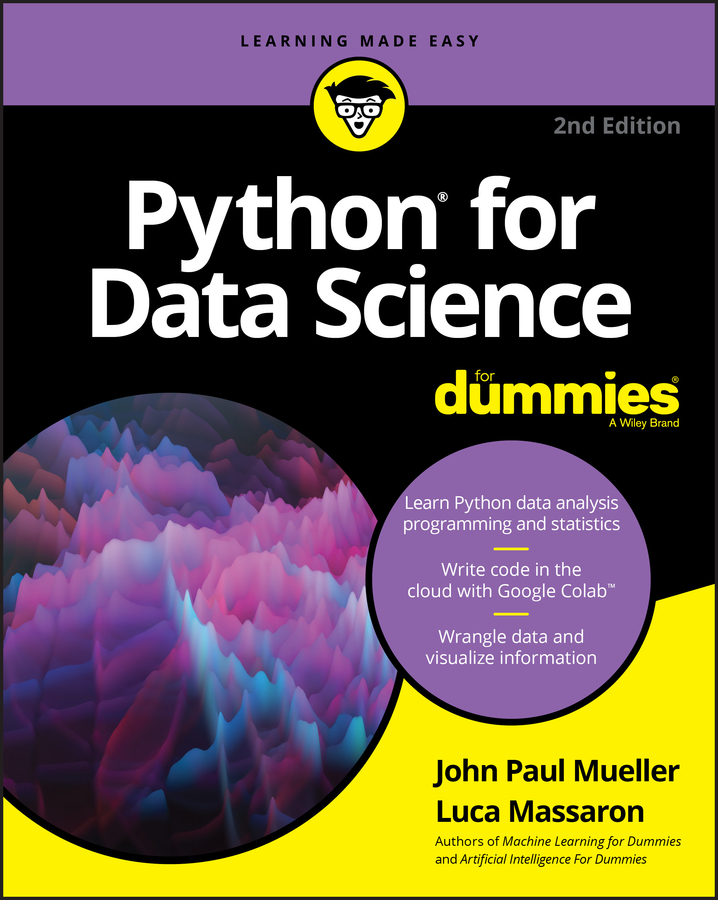##### Python for Data Science For DummiesData scientists can use Python to perform factor and principal component analysis. SVD operates directly on the numeric values in data, but you can also express data as a relationship between variables. Each feature has a certain variation. You can calculate the variability as the variance measure around the mean. The more the variance, the more the information contained inside the variable.

In addition, if you place the variable into a set, you can compare the variance of two variables to determine whether they correlate, which is a measure of how strongly they have similar values.

Checking all the possible correlations of a variable with the others in the set, you can discover that you may have two types of variance:

• Unique variance: Some variance is unique to the variable under examination. It cannot be associated to what happens to any other variable.

• Shared variance: Some variance is shared with one or more other variables, creating redundancy in the data. Redundancy implies that you can find the same information, with slightly different values, in various features and across many observations.

Of course, the next step is to determine the reason for shared variance. Trying to answer such a question, as well as determining how to deal with unique and shared variances, led to the creation of factor and principal component analysis.

## Considering the psychometric model

Long before many machine-learning algorithms were thought up, psychometrics, the discipline in psychology that is concerned with psychological measurement, tried to find a statistical solution to effectively measure dimensions in personality. The human personality, as with other aspects of human beings, is not directly measurable. For example, it isn’t possible to measure precisely how much a person is introverted or intelligent. Questionnaires and psychological tests only hint at these values.

Psychologists knew of SVD and tried to apply it to the problem. Shared variance attracted their attention: If some variables are almost the same, they should have the same root cause, they thought. Psychologists created factor analysis to perform this task! Instead of applying SVD directly to data, they applied it to a newly created matrix tracking the common variance, in the hope of condensing all the information and recovering new useful features called factors.

## Looking for hidden factors

A good way to show how to use factor analysis is to start with the Iris dataset.

```from sklearn.datasets import load_iris
from sklearn.decomposition import FactorAnalysis
X, y = iris.data, iris.target
factor = FactorAnalysis(n_components=4, , random_state=101).fit(X)```

After loading the data and having stored all the predictive features, the FactorAnalysis class is initialized with a request to look for four factors. The data is then fitted. You can explore the results by observing the components_ attribute, which returns an array containing measures of the relationship between the newly created factors, placed in rows, and the original features, placed in columns.

At the intersection of each factor and feature, a positive number indicates that a positive proportion exists between the two; a negative number, instead, points out that they diverge and one is the contrary to the other.

You’ll have to test different values of n_components because it isn’t possible to know how many factors exist in the data. If the algorithm is required for more factors than exist, it will generate factors with low values in the components_ array.

```import pandas as pd
print pd.DataFrame(factor.components_,columns=iris.feature_names)
sepal length (cm) sepal width (cm) petal length (cm) petal width (cm)
0   0.707227   -0.153147   1.653151   0.701569
1   0.114676   0.159763   -0.045604   -0.014052
2   0.000000   -0.000000   -0.000000   -0.000000
3   -0.000000   0.000000   0.000000   -0.000000```

In the test on the Iris dataset, for example, the resulting factors should be a maximum of 2, not 4, because only two factors have significant connections with the original features. You can use these two factors as new variables in your project because they reflect an unseen but important feature that the previously available data only hinted at.

## Using components, not factors

If an SVD could be successfully applied to the common variance, you might wonder why you can’t apply it to all the variances. Using a slightly modified starting matrix, all the relationships in the data could be reduced and compressed in a similar way to how SVD does it.

The results of this process, which are quite similar to SVD, are called principal components analysis (PCA). The newly created features are named components. In contrast to factors, components aren’t described as the root cause of the data structure but are just restructured data, so you can view them as a big, smart summation of selected variables.

For data science applications, PCA and SVD are quite similar. However, PCA isn’t affected by the scale of the original features (because it works on correlation measures that are all bound between -1 and +1 values) and PCA focuses on rebuilding the relationship between the variables, thus offering different results from SVD.

## Achieving dimensionality reduction

The procedure to obtain a PCA is quite similar to the factor analysis. The difference is that you don’t specify the number of components to extract. You decide later how many components to keep after checking the explained_variance_ratio_ attribute, which provides quantification of the informative value of each extracted component. The following example shows how to perform this task:

```from sklearn.decomposition import PCA
import pandas as pd
pca = PCA().fit(X)
print ‘Explained variance by component: %s’ % pca.explained_variance_ratio_
print pd.DataFrame(pca.components_,columns=iris.feature_names)
Explained variance by component: [ 0.92461621 0.05301557 0.01718514 0.00518309]
sepal length (cm) sepal width (cm) petal length (cm) petal width (cm)
0   0.361590   -0.082269   0.856572   0.358844
1   -0.656540   -0.729712   0.175767   0.074706
2   0.580997   -0.596418   -0.072524   -0.549061
3   0.317255   -0.324094   -0.479719   0.751121```

In this decomposition of the Iris dataset, the vector array provided by explained_variance_ratio_ indicates that most of the information is concentrated into the first component (92.5 percent). It’s therefore possible to reduce the entire dataset to just two components, providing a reduction of noise and redundant information from the original dataset.